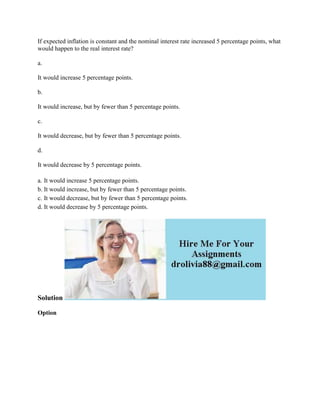Se está descargando tu SlideShare. ×

# If expected inflation is constant and the nominal interest rate increa (1).docx

Anuncio
Anuncio
Anuncio
Anuncio
Anuncio
Anuncio
Anuncio
Anuncio
Anuncio
Anuncio
AnuncioCargando en…3
×

1 de 2 Anuncio

# If expected inflation is constant and the nominal interest rate increa (1).docx

If expected inflation is constant and the nominal interest rate increased 5 percentage points, what would happen to the real interest rate?
a.
It would increase 5 percentage points.
b.
It would increase, but by fewer than 5 percentage points.
c.
It would decrease, but by fewer than 5 percentage points.
d.
It would decrease by 5 percentage points.
Solution
Option
.

If expected inflation is constant and the nominal interest rate increased 5 percentage points, what would happen to the real interest rate?
a.
It would increase 5 percentage points.
b.
It would increase, but by fewer than 5 percentage points.
c.
It would decrease, but by fewer than 5 percentage points.
d.
It would decrease by 5 percentage points.
Solution
Option
.

Anuncio
Anuncio

### If expected inflation is constant and the nominal interest rate increa (1).docx

1. 1. If expected inflation is constant and the nominal interest rate increased 5 percentage points, what would happen to the real interest rate? a. It would increase 5 percentage points. b. It would increase, but by fewer than 5 percentage points. c. It would decrease, but by fewer than 5 percentage points. d. It would decrease by 5 percentage points. a. It would increase 5 percentage points. b. It would increase, but by fewer than 5 percentage points. c. It would decrease, but by fewer than 5 percentage points. d. It would decrease by 5 percentage points. Solution Option